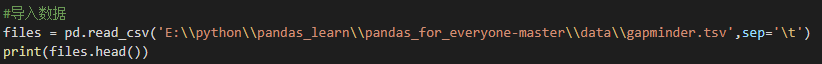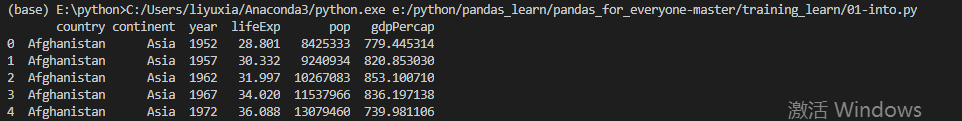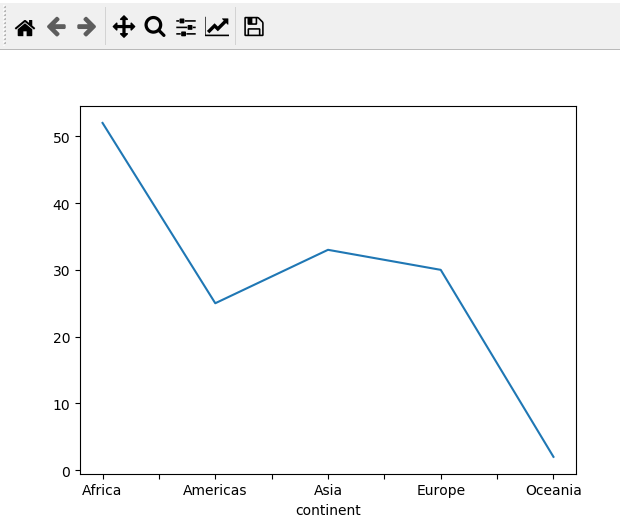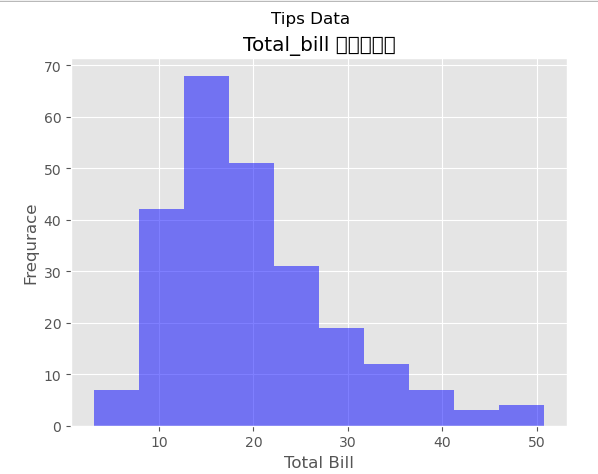# pandas ~ 基础 pandas### 二：pandas 的常用功能

##### 1、pandas导入数据以及查看数据的类型

import pandas as pd (一般简写，别人都这么些)##### 2、导入数据后就可以进行对数据和数据结构的查询

print(type(df)) 就是使用type()函数来看df 的类型

print(df.shape) 就是查看df 的结构类型

print(df.dtypes)就是查看每一列的数据类型

print(df.columns)就是查看数据的列的名称

print(df.info()) 使用info函数查看数据的每一列的数据的类型和空值等情况。

#files 中加载了数据可以看看数据的属性print(type(files))#可以看看数据的结构,其中shape 代表是属性，所以不需要括号print(files.shape)#当然也可以查看数据的每个列的属性,同样的dtypes也是属性print(files.dtypes)#查看数据的列的名称print(files.columns)#使用info()函数来获得数据的详细信息print(files.info())

3、数据的运算

#如果你导入了我的数据，那么你应该操作和我一样的#我们按照不同年份对pop进行求平均year_by_pop = files.groupby('year')['pop'].mean()#当然有时候不仅仅是简单的一个维度，也可能是多了维度，按照国家和年的形式year_country_by_pop = files.groupby(['year','country'])['pop'].mean()#我们也可以进行按照州的形式对国家进行计数计算continent_country_count = files.groupby('continent')['contry'].nunique()
##### 3、matplotlib的形式查看图

import matplotlib.pyplot as plt

#先导入数据的包import matplotlib.pyplot as pltcontinent_country_count.plot()plt.show()#这样一个简单的图就画好了

﻿### 三：使用matplotlib 绘制简单的图形

##### 1、matplotlib 绘制的套路

#使用matplotlib进行作图import matplotlib.pyplot as pltimport seaborn as sns    #导入你需要的数据，这里使用sns 中的tips 数据tips = sns.load_dataset('tips')print(tips.head())print(tips.describe())print(tips.info())print(tips.columns)print(tips.shape)#可以设置对应图形的styple plt.styple.use('ggplot')#可以使用以上语句对数据做简单的探索#使用其中的totle_bil做离散型数据展示hist = plt.figure()#创建轴域axis1 =hist.add_subplot(1,1,1)#然后绘制直方图axis1.hist(tips['total_bill'],bins=10)#然后绘制对应的标题axis1.set_title('Hist of Total_bill')axis1.set_xlabel('Total_bill')axis1.set_ylabel('Frequare')#展示图形plt.show()

﻿axis1.scatter(x,y) #用于两个连续变量

axis1.boxplot(数据，labels )

axis1.plot(x,y)

﻿## 评论